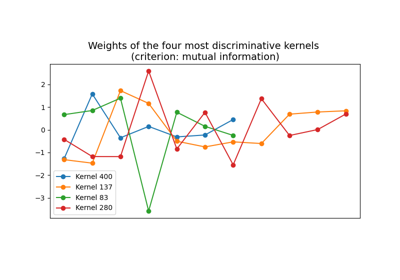# `pyts.transformation`.ROCKET¶

class `pyts.transformation.``ROCKET`(n_kernels=10000, kernel_sizes=(7, 9, 11), random_state=None)[source]

RandOm Convolutional KErnel Transformation.

This algorithm randomly generates a great variety of convolutional kernels and extracts two features for each convolution: the maximum and the proportion of positive values.

Parameters: n_kernels : int (default = 10000) Number of kernels. kernel_sizes : array-like (default = (7, 9, 11)) The possible sizes of the kernels. random_state : None, int or RandomState instance (default = None) The seed of the pseudo random number generator to use when shuffling the data. If int, random_state is the seed used by the random number generator. If RandomState instance, random_state is the random number generator. If None, the random number generator is the RandomState instance used by np.random.

References

  A. Dempster, F. Petitjean and G. I. Webb, “ROCKET: Exceptionally fast and accurate time series classification using random convolutional kernels”. https://arxiv.org/abs/1910.13051.

Examples

```>>> from pyts.transformation import ROCKET
>>> X = np.arange(100).reshape(5, 20)
>>> rocket = ROCKET(n_kernels=10)
>>> rocket.fit_transform(X).shape
(5, 20)
```
Attributes: weights_ : array, shape = (n_kernels, max(kernel_sizes)) Weights of the kernels. Zero padding values are added. length_ : array, shape = (n_kernels,) Length of each kernel. bias_ : array, shape = (n_kernels,) Bias of each kernel. dilation_ : array, shape = (n_kernels,) Dilation of each kernel. padding_ : array, shape = (n_kernels,) Padding of each kernel.

Methods

 `__init__`([n_kernels, kernel_sizes, random_state]) Initialize self. `fit`(X[, y]) Fit the model according to the given training data. `fit_transform`(X[, y]) Fit to data, then transform it. `get_params`([deep]) Get parameters for this estimator. `set_params`(**params) Set the parameters of this estimator. `transform`(X) Transform the provided data.
`__init__`(n_kernels=10000, kernel_sizes=(7, 9, 11), random_state=None)[source]

Initialize self. See help(type(self)) for accurate signature.

`fit`(X, y=None)[source]

Fit the model according to the given training data.

Parameters: X : array-like, shape = (n_samples, n_timestamps) Training vector. y : None or array-like, shape = (n_samples,) Class labels for each data sample. Ignored. self : object
`fit_transform`(X, y=None, **fit_params)

Fit to data, then transform it.

Fits transformer to X and y with optional parameters fit_params and returns a transformed version of X.

Parameters: X : array-like, shape = (n_samples, n_timestamps) Univariate time series. y : None or array-like, shape = (n_samples,) (default = None) Target values (None for unsupervised transformations). **fit_params : dict Additional fit parameters. X_new : array Transformed array.
`get_params`(deep=True)

Get parameters for this estimator.

Parameters: deep : bool, default=True If True, will return the parameters for this estimator and contained subobjects that are estimators. params : dict Parameter names mapped to their values.
`set_params`(**params)

Set the parameters of this estimator.

The method works on simple estimators as well as on nested objects (such as `Pipeline`). The latter have parameters of the form `<component>__<parameter>` so that it’s possible to update each component of a nested object.

Parameters: **params : dict Estimator parameters. self : estimator instance Estimator instance.
`transform`(X)[source]

Transform the provided data.

Parameters: X : array-like, shape = (n_samples, n_timestamps) Test samples. X_new : array, shape = (n_samples, 2 * n_kernels) Extracted features from the kernels.

## Examples using `pyts.transformation.ROCKET`¶RandOm Convolutional KErnel Transform (ROCKET)